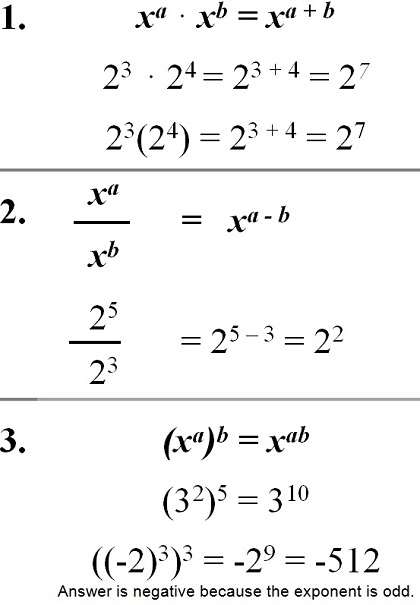# Laws of Exponents

Back to “Exponents” Page

Familiarize with these basic laws. Then, use the flashcards below to practice them and increase your speed and stamina using them.Use Rule #1 above for these flash cards.

• ﻿xa · xb = ; xa + b
• 22 · 2 = ; 22 + 1 = 23 = 8
• 22 · 22 = ; 22 + 2 = 24 = 16
• 22 · 23 = ; 22 + 3 = 25 = 32
• 22 · 24 = ; 22 + 4 = 26 = 64
• 23 · 24 = ; 23 + 4 = 27 = 128
• 23 · 25 = ; 23 + 5 = 28 = 256
• 23 · 26 = ; 23 + 6 = 29 = 512
• 23 · 27 = ; 23 + 7 = 210 = 1024
• 32 · 32 = ; 32 + 2 = 34 = 81
• 32 · 33 = ; 32 + 3 = 35 = 243
• 33 · 33 = ; 33 + 3 = 36 = 729

Use Rule #2 above for these flash cards.

• ﻿xa / xb = ; xa - b
• 23 / 2 = ; 23 - 1 = 22 = 4
• 26 / 23 = ; 26 - 3 = 23 = 8
• 28 / 23 = ; 28 - 3 = 25 = 32
• 36 / 32 = ; 36 - 2 = 34 = 81
• 38 / 33 = ; 38 - 3 = 35 = 243
• 38 / 32 = ; 38 - 2 = 36 = 729
• 39 / 32 = ; 39 - 2 = 37 = 2,187

Use Rule #3 above for these flash cards.

• ﻿(xa)b = ; xab
• (22)2 = ; 22 × 2 = 24 = 16
• (22)3 = ; 22 × 3 = 26 = 64
• (24)2 = ; 24 × 2 = 28 = 256
• (23)3 = ; 23 × 3 = 29 = 512
• (22)5 = ; 22 × 5 = 210 = 1024
• (32)2 = ;34 = 81
• (32)3 = ;36 = 729
• (52)2 = ;54 = 625
• (52)3 = ;56 = 15,625